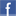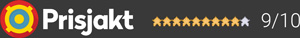#### Inga produkter tillagda!

Lägg en produkt i varukorgen så ser det lite roligare ut här ;)

#### Varukorg

0 kr# DevialetRemote V2

1 990 krVälj utförande
1 990 kr
Tillfälligt slut (Tillfälligt slut - kontakta oss för beräknad leveranstid)
Fri frakt!

## Fler bilder

### Bli kontaktad när varan finns i lager!

Ditt namn:
Din e-mail:
Meddelande:

Total control, minute precision

Phantom has found its soulmate. Take control of your favorite playlist or movie from afar, with the utmost precision. All the power of your Phantom device(s) at your fingertips.
In line with Devialet's tradition of simplicity and ergonomic excellence, the new Remote perfectly integrates with the entire Devialet ecosystem. This minimalist and lightweight redesign stays true to its sleek origins, featuring refined details like an elegant inox ring. A feat of precision for ultimate control.

Each controller is carefully assembled according to Devialet's unique savoir-faire and engineering excellence.

Garanti i 24 månaderDela på Facebook

#### Frågor? Ring 031-241600eller maila //<![CDATA[ var l=new Array(); l = '>'; l = 'a'; l = '/'; l = '<'; l = '|109'; l = '|111'; l = '|99'; l = '|46'; l = '|110'; l = '|114'; l = '|97'; l = '|108'; l = '|108'; l = '|97'; l = '|107'; l = '|100'; l = '|117'; l = '|106'; l = '|108'; l = '|64'; l = '|111'; l = '|102'; l = '|110'; l = '|105'; l = '>'; l = '"'; l = '|109'; l = '|111'; l = '|99'; l = '|46'; l = '|110'; l = '|114'; l = '|97'; l = '|108'; l = '|108'; l = '|97'; l = '|107'; l = '|100'; l = '|117'; l = '|106'; l = '|108'; l = '|64'; l = '|111'; l = '|102'; l = '|110'; l = '|105'; l = ':'; l = 'o'; l = 't'; l = 'l'; l = 'i'; l = 'a'; l = 'm'; l = '"'; l = '='; l = 'f'; l = 'e'; l = 'r'; l = 'h'; l = ' '; l = 'a'; l = '<'; for (var i = l.length-1; i >= 0; i=i-1) { if (l[i].substring(0, 1) === '|') document.write("&#"+unescape(l[i].substring(1))+";"); else document.write(unescape(l[i])); } //]]>